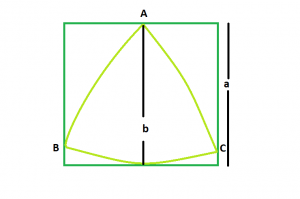# Biggest Reuleaux Triangle within A Square

Given an integer a which is the side of a square, the task is to find the biggest Reuleaux Triangle that can be inscribed within it.Examples:

Input: a = 6
Output: 25.3717

Input: a = 8
Output: 45.1053

## Recommended: Please try your approach on {IDE} first, before moving on to the solution.

Approach: We know that the Area of Reuleaux Traingle is 0.70477 * b2 where b is the distance between the parallel lines supporting the Reuleaux Triangle.
From the figure, it is clear that distance between parallel lines supporting the Reuleaux Triangle = Side of the square i.e. a
So, Area of the Reuleaux Triangle, A = 0.70477 * a2

Below is the implementation of the above approach:

## C++

 `// C++ Program to find the area ` `// of the biggest Reuleaux triangle ` `// that can be inscribed within a square ` `#include ` `using` `namespace` `std; ` ` `  `// Function to find the Area ` `// of the Reuleaux triangle ` `float` `ReuleauxArea(``float` `a) ` `{ ` ` `  `    ``// Side cannot be negative ` `    ``if` `(a < 0) ` `        ``return` `-1; ` ` `  `    ``// Area of the Reuleaux triangle ` `    ``float` `A = 0.70477 * ``pow``(a, 2); ` `    ``return` `A; ` `} ` ` `  `// Driver code ` `int` `main() ` `{ ` `    ``float` `a = 6; ` `    ``cout << ReuleauxArea(a) << endl; ` `    ``return` `0; ` `} `

## Java

 `// Java Program to find the area ` `// of the biggest Reuleaux triangle ` `// that can be inscribed within a square ` `import` `java.lang.Math; ` `class` `cfg  ` `{ ` `// Function to find the Area ` `// of the Reuleaux triangle ` ` ``static` `double` `ReuleauxArea(``double` `a) ` `{ ` ` `  `    ``// Side cannot be negative ` `    ``if` `(a < ``0``) ` `        ``return` `-``1``; ` ` `  `    ``// Area of the Reuleaux triangle ` `    ``double` `A = ``0.70477` `* Math.pow(a, ``2``); ` `    ``return` `A; ` `} ` ` `  `// Driver code ` `public` `static` `void` `main(String[] args) ` `{ ` `    ``double` `a= ``6``; ` `    ``System.out.println(ReuleauxArea(a) ); ` `     `  `} ` `}``//This code is contributed by Mukul Singh.  `

## Python3

 `# Python3 Program to find the area  ` `# of the biggest Reuleaux triangle  ` `# that can be inscribed within a square  ` ` `  `# Function to find the Area  ` `# of the Reuleaux triangle  ` `def` `ReuleauxArea(a) : ` ` `  `    ``# Side cannot be negative  ` `    ``if` `(a < ``0``) : ` `        ``return` `-``1` ` `  `    ``# Area of the Reuleaux triangle  ` `    ``A ``=` `0.70477` `*` `pow``(a, ``2``);  ` `    ``return` `A ` ` `  `# Driver code  ` `if` `__name__ ``=``=` `"__main__"` `: ` ` `  `    ``a ``=` `6` `    ``print``(ReuleauxArea(a))  ` ` `  `# This code is contributed by Ryuga `

## C#

 `// C# program to find area of the  ` `//biggest  Reuleaux triangle that can be inscribed ` `//within a square ` `using` `System;  ` `   `  `class` `GFG {  ` `   `  `    ``// Function to find the area  ` `    ``// of the reuleaux triangle  ` `    ``static` `double` `reuleauxArea(``double` `a)  ` `    ``{  ` `   `  `     ``//Side cannot be negative ` `     ``if` `(a<0) ` `        ``return` `-1; ` `         `  `        ``// Area of the reauleaux triangle  ` `        ``double` `A=0.70477*Math.Pow(a,2); ` `        ``return` `A; ` `    ``}  ` `   `  `    ``// Driver code  ` `    ``static` `public` `void` `Main()  ` `    ``{  ` `        ``double` `a= 6;  ` `        ``Console.WriteLine(reuleauxArea( a));  ` `    ``}  ` `}  ` `//This code is contributed by Mohit kumar 29 `

## PHP

 ` `

Output:

```25.3717
```

Attention reader! Don’t stop learning now. Get hold of all the important DSA concepts with the DSA Self Paced Course at a student-friendly price and become industry ready.

My Personal Notes arrow_drop_upCheck out this Author's contributed articles.

If you like GeeksforGeeks and would like to contribute, you can also write an article using contribute.geeksforgeeks.org or mail your article to contribute@geeksforgeeks.org. See your article appearing on the GeeksforGeeks main page and help other Geeks.

Please Improve this article if you find anything incorrect by clicking on the "Improve Article" button below.